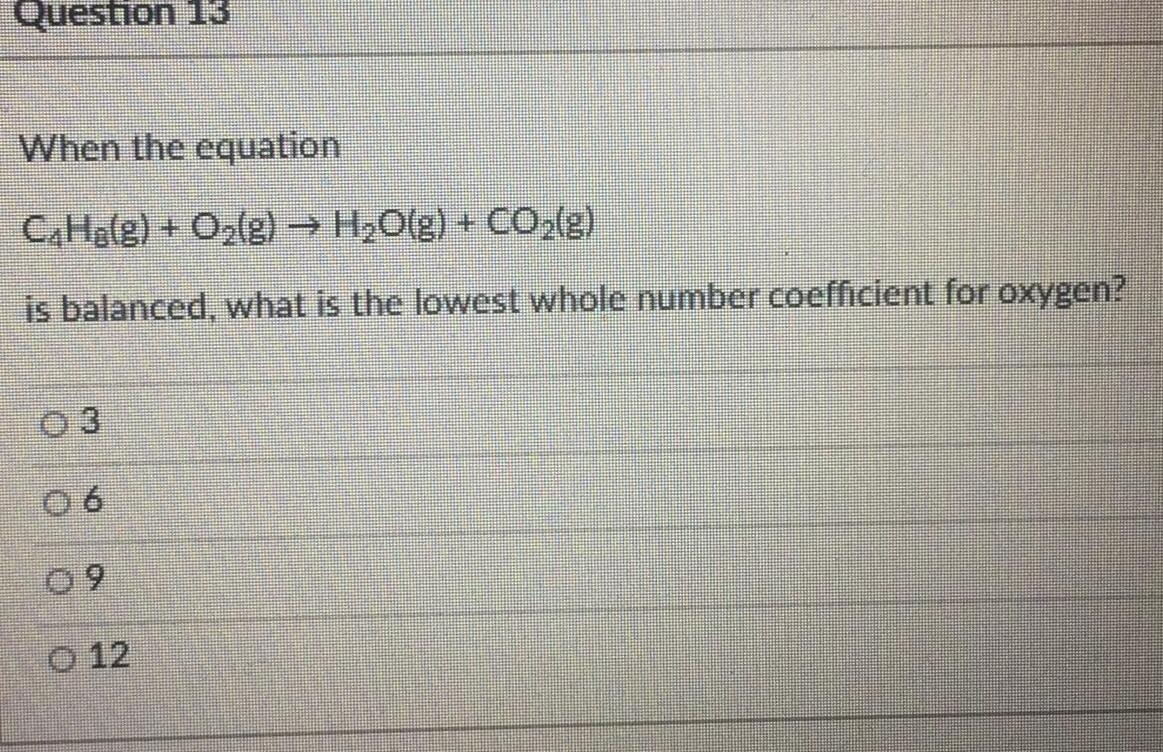Question:

# When the equation C₂H₂(g) + O₂(g) → H₂O(g) + CO₂(g) is

Last updated: 8/8/2022When the equation C₂H₂(g) + O₂(g) → H₂O(g) + CO₂(g) is balanced, what is the lowest whole number coefficient for oxygen?